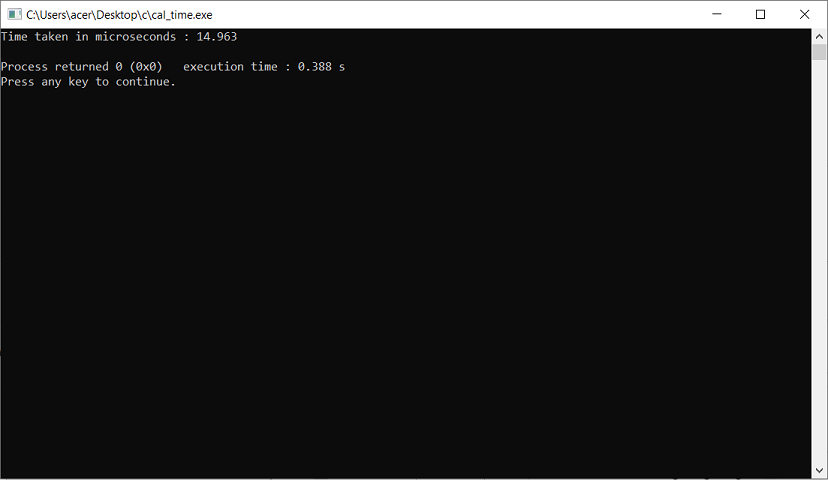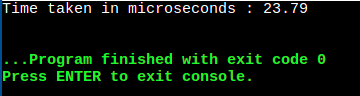GeeksforGeeks App
Open AppBrowser
Continue

# How to Calculate Running Time in Microseconds in C++?

Prerequisite: Chrono Library in C++

The task is to calculate the running time of a program in Microseconds in C++

`#include<chrono>`

The Chrono library handles operations relating to time and date. This library deals with the fact that timers and clocks might be different on different systems and thus improve over time in terms of precision.

Chrono is both a header file and even a namespace. All the elements in this header (except for the common_type specializations) are not defined directly under the std namespace (like most of the standard library) but under the std::chrono namespace.

### Use of duration_cast:

Here function_performing_iterations() is a dummy function that iterates from i=1 to i=10^7. Now we start counting time by writing

`auto duration = duration_cast<microseconds>(stop1 - start1);`

Now when the compiler executes the function-> function_performing_iterations(), the control then again comes back to main(), after which the time is stopped. (For reference it can be assumed as a race i.e. when in a race as soon as the runners start running, the timer is started and when they reach the finish line, the timer is stopped)

Now the time is calculated as:

`auto duration = duration_cast<microseconds>(stop1 - start1);`

Finally, the time taken in microseconds is printed by type casting in double because the a/b normal division returns an integer value.

Example:

## C++

 `// C++ to calculate time in microseconds``#include ``#include ` `using` `namespace` `std;` `// this namespace is used``// to perform calculation``using` `namespace` `chrono;` `// A function performing 10^7 operations``void` `function_performing_iterations()``{``    ``for` `(``int` `i = 0; i < 10000000;) {``        ``i++;``    ``}``}` `//  Driver Code``int` `main()``{``    ``// Timer starts now``    ``auto` `start1 = high_resolution_clock::now();` `    ``function_performing_iterations();` `    ``// Timer ends now``    ``auto` `stop1 = high_resolution_clock::now();``    ``// Difference is calculated``    ``auto` `duration``        ``= duration_cast(stop1 - start1);` `    ``// Displays time in output file in``    ``// microseconds``    ``cerr << ``"Time taken in microseconds : "``         ``<< (``double``)(duration.count() / 1000.0) << endl;``}`

Output:

`Time taken in microseconds : 14.963`### By using the Boost library

Boost Libraries are intended to be widely useful, and usable across a broad spectrum of applications. For example, they are helpful for handling large numbers having a range beyond the long long, long double data type (264) in C++.

Installation:

Please refer to this Article for the installation of the boost. We can download the zip file. After that, we just need to extract the whole in a specified folder of GCC or we can do this easily by command prompt.

Example:

## C++

 `// Cpp to calculate``// Running time in Microseconds` `#include ``using` `namespace` `boost::multiprecision;``using` `namespace` `std;`  `int` `main() {`  `    ``// The starting time before performing the task``    ``boost::posix_time::ptime start = boost::posix_time::second_clock::local_time();`   `    ``for` `(``int` `i = 1; i <= 10000000; i++)``    ``{``        ``//Performing Random task ...iterations from 1 to 10^7``    ``}`  `    ``// Getting the current time after executing Task` `    ``boost::posix_time::ptime end = boost::posix_time::second_clock::local_time();` `    ``// Calculating the difference by performing (end time - start time)` `    ``boost::posix_time::time_duration dur = end - start;`  `    ``//Calculation time in microseconds` `    ``std::cout << ``"Time Diff in Total Micro Seconds "` `<< dur.total_microseconds() << std::endl;` `}`

Output:My Personal Notes arrow_drop_up